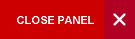## Fluid Dynamics

Semester 1 and 2

This course focuses on basic principles in fluid dynamics. Basic mathematical concepts are reviewed and used to analyze the kinematics and dynamics of fluid motions (e.g. . solving problems related to pipe flow, energy delivery by propellers, energy extraction by turbines, modeling of blood flow in circulatory systems , and atmospheric flows).

Syllabus:

Introduction to Mathematical Concepts in Fluid Dynamics:

• Vector analysis and basic mathematical tools;
• physical characteristics of the fluid state and description of flow types;
• viscosity coefficients as they relate to laminar and turbulent flows;
• the Poiseuille equation

Kinematics and Dynamics of Fluid Motion:

• In-compressible and compressible fluids;
• Euler’s equations of motion;
• Bernoulli’s equation and its application;
• continuity equation;
• analyses of steady fluid flow, propeller, wind turbine, and wind velocity profile;
• Navier-Stokes equation and descriptions of boundary layer and turbulence;
• vertical transport of kinetic energy, mass, heat, moisture and pollutants.

Introduction to Atmospheric flows:

• Apparent forces (Coriolis and centrifugal) in rotating coordinate systems and their effects;
• geostrophic flows;
• qualitative introduction to Ekman layer;
• basic treatment of Rossby waves and Kelvin waves
Evaluation:

The course assessment will be conducted as follows:

Coursework

Two 1-hour in-course tests (equal weighting)      40%

Final Examination

One 2- hour final written examination                60%

Students will be expected to satisfy the examiners in both components.

Learning Objectives:

At the end of the course, students should be able to:

• use vector analysis to derive surface and volume integrals, and apply them to solve problems in basic fluid mechanics
• apply the Poiseuille equation to analyze the blood flow mechanism in the human circulatory system
• describe the basic principles governing the operation of propellers, wind turbines, hydro- power systems, and determine their energy efficiencies
• describe the concept of the ‘boundary layer’ and its application to turbulence and transport processes
• solve problems of  turbulent motion in various liquid and gaseous
• analyse atmospheric flows using the mathematical tools applicable to rotating coordinate systems
PHYS1411 and PHYS1412 and PHYS1421 and PHYS1422

Prescribed

• Douglas, J. F. Gasiorek, J. M. , Swaffield, J. A., and  Jack L.B., (2011),  Fluid Mechanics, 6th Edition; Prentice Hall, ISBN 978-0-273-71772-0

Highly Recommended

• Massey, B. S. and Ward-Smith, J. (2011), Mechanics of Fluids, 9th Edition; CRC Press. ISBN-10: 0415602602; ISBN-13: 978-0415602600

Online Resources:

Course Code:
PHYS2671
Credits:
3 Credits
Level:
Level 2
Top of Page